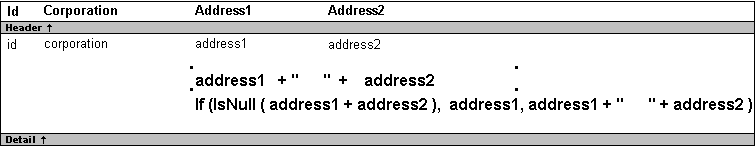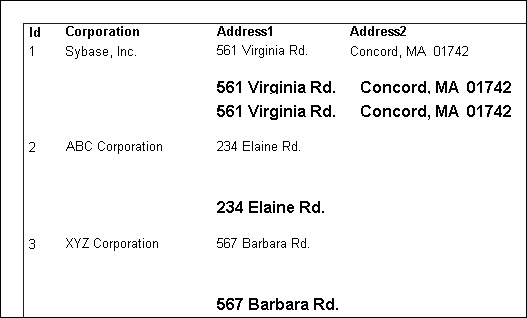## Four examples

The following topics provide examples that illustrate using DataWindow expression functions.

### Example 1: counting null values in a column

A null value is a marker used to fill a place in a column where data is missing for any reason. The value might not be applicable, or it might be missing or unknown. When a database table is created, each column in the table either allows null values or does not allow them. The column or set of columns that define the primary key cannot allow null values. Sometimes it is useful to know how many null values there are in a particular column.

What you want to do

Suppose you are working with the Fin_code table in the Enterprise Application Sample Database. The Fin_code table has three columns:

Column

What the column is

Allows null values?

Code

Unique financial identifier (primary key)

No

Type

Code type: expense or revenue

No

Description

Code description: the department incurring the expense or getting the revenue

Yes

You create a DataWindow object using the Code and Description columns. You want to know the number of null values in the Description column.

How to do it

In the DataWindow object, you create a computed field that uses functions to display the number of null values in the Description column.

For the sake of demonstrating the use of functions, the following computed fields are created in the Summary band of the DataWindow object (with text objects that tell you what information each computed field is providing):

`Count(description for all)`

counts the number of descriptions (that are not null);

`Sum(If(IsNull(description), 1, 0))`

returns a 1 if the description column is null, a 0 if the description column is not null, and then adds the total;

`Count(id for all)`

counts the number of IDs (which is also the number of rows);

`Sum(If(IsNull(description), 1, 1))`

adds the number of nulls and not nulls in the description column (which is the total number of rows) and should match the result of the

Count( id for all ) function; and

`IsNull(description)`

evaluates whether the last row in the table has a description that is null. The return value of the IsNull function is true or false.

What you get

Here is the design for the DataWindow object.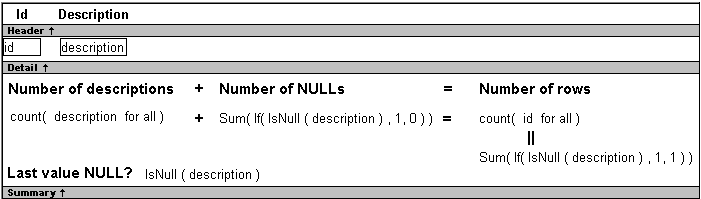Here is the DataWindow object showing eight descriptions, three of which are null and five of which are not null. The last description for Id=8 is null.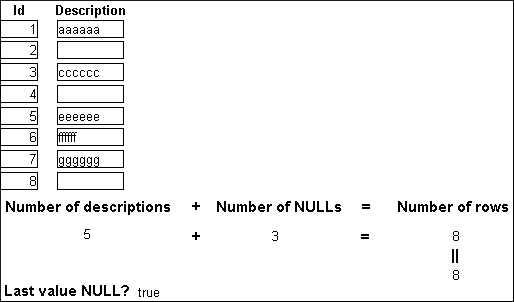### Example 2: counting active and terminated employees

Example 1 demonstrates the use of the Sum and Count functions. Sum and Count are two examples of a class of functions called aggregate functions.

An aggregate function is a function that operates on a range of values in a column. The aggregate functions are:

 Avg Large Mode Sum Count Last Percent Var CumulativePercent Max Small VarP CumulativeSum Median StDev First Min StDevP

Although the crosstab functions (CrosstabAvg, CrosstabAvgDec, CrosstabCount, CrosstabMax, CrosstabMaxDec, CrosstabMin, CrosstabMinDec, CrosstabSum, and CrosstabSumDec) behave like aggregate functions, they are not included on the list because they are for crosstabs only and are designed to work in the crosstab matrix.

A few restrictions apply to the use of aggregate functions. You cannot use an aggregate function:

• In a filter

• In a validation rule

• As an argument for another aggregate function

This example demonstrates the use of the Sum aggregate function.

What you want to do

Using the employee table in the PB Demo DB as the data source, you create a DataWindow object using at least the Emp_id and the Status columns. You want the DataWindow object to display the number of active employees, terminated employees, and on leave employees in the company.

How to do it

In the summary band in the workspace, add three computed fields to the DataWindow object that use the Sum and If functions:

`Sum(If(status = "A", 1, 0))`

counts the number of Active employees in your company;

`Sum(If(status = "T", 1, 0))`

counts the number of Terminated employees in your company;

`Sum(If(status = "L",1,0))`

counts the number of On Leave employees in your company.

By clicking the Page computed field button, you can also add a Page computed field in the footer band to display the page number and total pages at the bottom of each page of the DataWindow object.

What you get

Here is what the design of the DataWindow object looks like.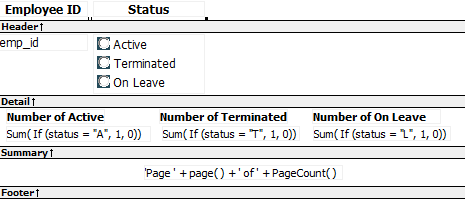Here is the last page of the DataWindow object, with the total number of active employees, terminated employees, and on leave employees in the company displayed.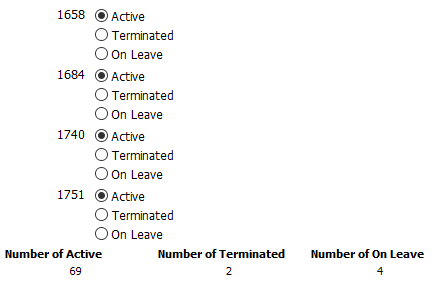What if you decide that you also want to know the number of active employees, terminated employees, and on leave employees in each department in the company?

To display the active employees, terminated employees, and on leave employees in each department:

1. Select Design>Data Source from the menu bar so that you can edit the data source.

2. Select Design>Select tables from the menu bar and open the Department table in the Select painter workspace, which currently displays the Employee table with the Emp_id and Status columns selected.

3. Select the department_dept_name column to add it to your data source.

4. Select Rows>Create Group from the menu bar to create a group and group by department name.

`Sum(If(status = "A", 1, 0) for group 1)`

counts the number of active employees in each department;

`Sum(If(status = "T", 1, 0) for group 1)`

counts the number of terminated employees in each department;

`Sum(If(status = "L", 1, 0) for group 1)`

counts the number of on leave employees in each department.

Here is what the design of the grouped DataWindow object looks like.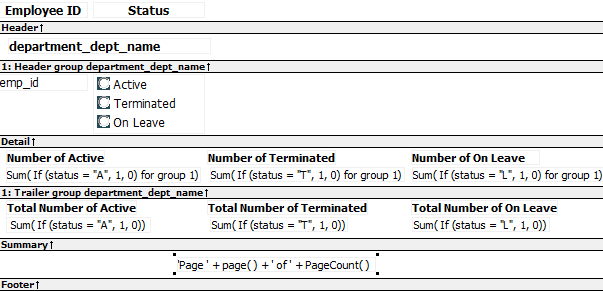Here is the last page of the DataWindow object with the number of active employees, terminated employees, and on leave employees in the shipping department displayed, followed by the total number of active employees, terminated employees, and on leave employees in the company.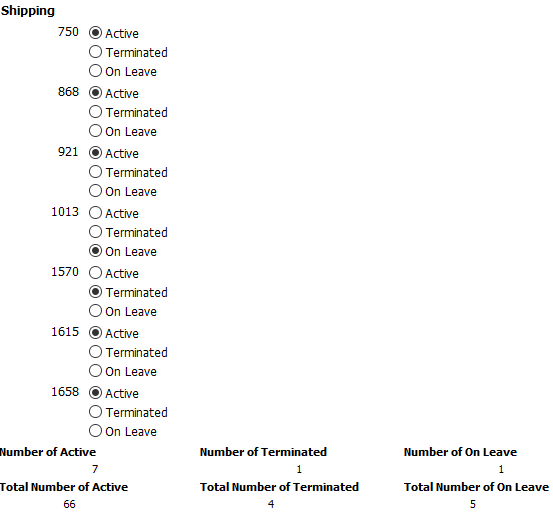### Example 3: creating a row indicator

This example demonstrates the use of several functions: Bitmap, Case, CurrentRow, GetRow, and RGB.

What you want to do

Using the Employee table in the Enterprise Application Sample Database, you create a DataWindow object using the Emp_id, Emp_fname, Emp_lname, and Salary columns.

In the painter, you want to display a number of items such as the number of the current row, an arrow that is an indicator of the current row, and the salary for an employee with a background color that depends on what the salary is.

How to do it

In the workspace, add the following:

• A computed field CurrentRow( ), which displays the number of the current row.

• A picture object, which is a right-arrow, for which you define an expression for the arrow's visible property:

`If(CurrentRow()= GetRow(), 1, 0)`

The expression causes an arrow to display in the current row and no arrow to display in other rows.

• A computed field using the If, CurrentRow, and GetRow functions:

`If(CurrentRow() = GetRow(),"Current","Not current")`

displays the word "Current" when the row is the current row and "Not current" for all other rows.

• A computed field (typed on one line) using the Bitmap, CurrentRow, and GetRow functions:

`Bitmap(If(CurrentRow()= GetRow(), "c:\sampl\ex\code\indicatr.bmp", " "))`

displays an arrow bitmap for the current row and no bitmap for all other rows.

• An expression for the Background.Color property of the salary column:

```Case(salary WHEN IS >60000 THEN RGB(192,192,192)
WHEN IS >40000 THEN RGB(0,255,0) ELSE
RGB(255,255,255))```

The expression causes a salary above \$40,000 to display in green, a salary above \$60,000 to display in gray, and all other salaries to display in white.

What you get

Here is what the design of the DataWindow object looks like: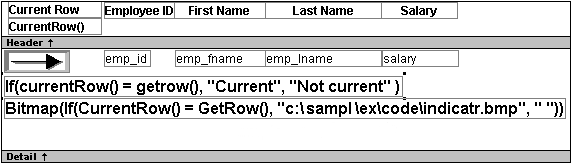Here is what the data looks like with the second row current.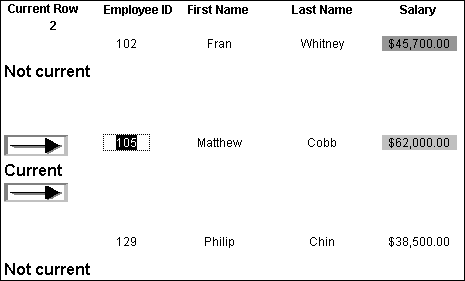Notice that the number of the current row is 2; the first row and the third row are "Not current" (and therefore display no bitmap); and the second row, which is the current row, displays the arrow row indicator.

On your screen, the salary in the first row has a green background because it is more than \$40,000; the salary in the second row has a gray background because it is more than \$60,000; and the salary in the third row has a white background, which matches the background of the DataWindow object.

### Example 4: displaying all data when a column allows nulls

When you create an arithmetic expression that has a null value, the value of the expression is null. This makes sense, since null means essentially undefined and the expression is undefined, but sometimes this fact can interfere with what you want to display.

What you want to do

A table in your database has four columns: Id, Corporation, Address1, and Address2. The Corporation, Address1, and Address2 columns allow null values. Using this table as the data source, you create a DataWindow object using the four columns. You now want the DataWindow object to display both parts of the address, separated by a comma.

You create a computed field to concatenate Address1 and Address2 with a comma separator. Here is the expression that defines the computed field:

`address1 + ", " + address2`

When you preview the DataWindow object, if either Address1 or Address2 is null, no part of the address displays because the value of the expression is null. To display a part of the address, you need to create a computed field that forces evaluation even if Address2 is null. Note that Address2 is assumed to have data only if Address1 has data for a particular row.

How to do it

In the detail band, create a computed field that uses the If and IsNull functions:

```If(IsNull(address1 + address2), address1, address1